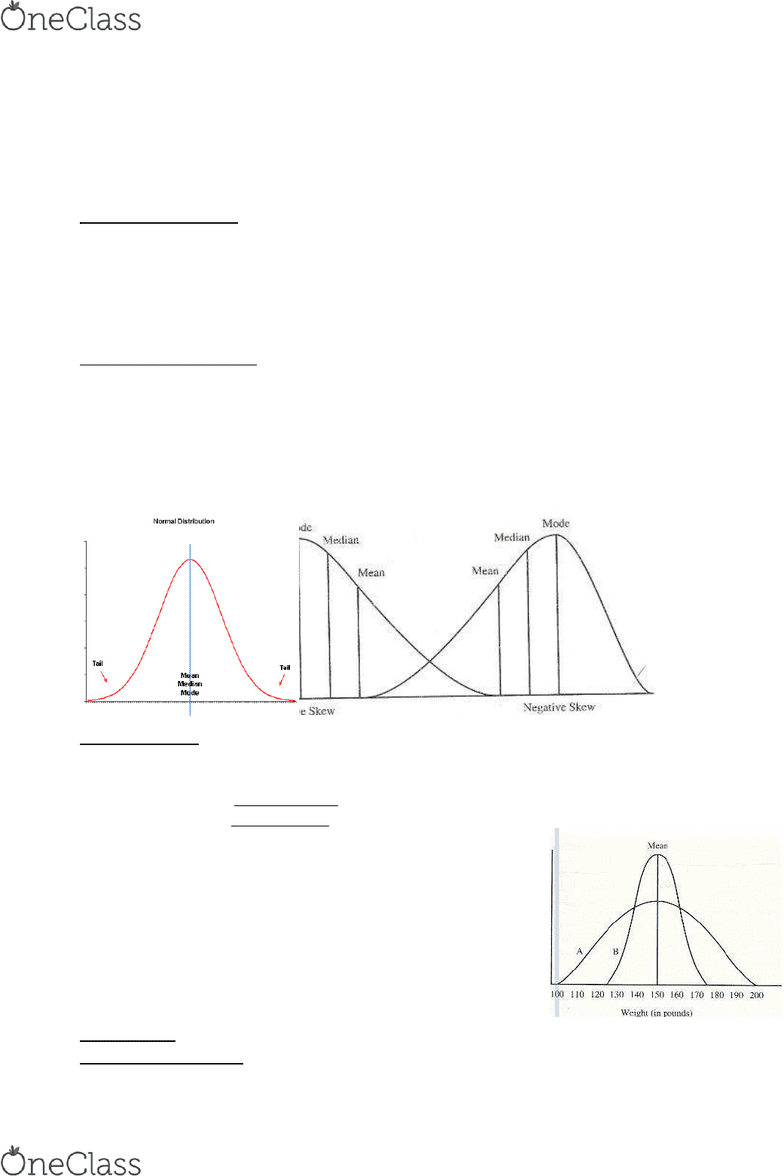Textbook Notes (280,000)
CA (170,000)
UOttawa (6,000)
HSS (300)
Chapter 3

# HSS 2381 Chapter Notes - Chapter 3: Central Tendency, Xm Satellite Radio, Descriptive Statistics

Department
Health Sciences
Course Code
HSS 2381
Professor
Eleanor Riesen
Chapter
3

This preview shows pages 1-2. to view the full 8 pages of the document.Chapter 3: Central Tendency Variability, and Relative Standing
- Both central tendency and variability can be expressed by indexes that are descriptive statistics
- Central Tendency: refers to location of typical data value which cluster around another variable
- Indexes of central tendency provide a single number to characterize a distribution
- Central tendency measures come from distribution center of data values = indicate "typical" where
data values cluster
- Popularly called an average
A) Central Tendency Indexes => 3 alternative indexes:
1. The mode
2. The median
3. The mean
1) The Mode: the soe alue that ous ost feue; the ost popula soe
- Ex: Age: 26 27 27 28 29 30 31 = Mode = 27
1. Can be used with data measured on any measurement level (including nominal level)
2. Eas to opute
3. Reflects an actual value in the distribution, = easy to understand
4. Useful he thee ae + popula soes i ultiodal distiutios
1. Ignores most information in the distribution
2. Tends to be unstable (value varies a lot from one sample to the next)
3. Some distributions may not have a mode (ex = 10, 10, 11, 11, 12, 12)
2) The Median: point that divides the distribution into equal half; 50% are below median & 50% above
Ex: Age: 26 27 27 28 29 30 31 = Median (Mdn) = 28
1. Not influenced by outliers
2. Patiulal good ide of hat is tpial he distiutio is skeed
3. Eas to opute
4. Appropriate for ordinal level
1. Doesn't take actual data values into accountonly an index of position
2. Value of median not necessarily an actual data value = more difficult to understand vs mode
3) The Mean: the arithmetic average & most commonly used; Data values are summed & divided by N
Ex: Age: 26 27 27 28 29 30 31 => Mean = 28.3
- Preferred for interval- & ratio-level data
Equation:
M = ΣX ÷ N
Where:
M / X bar = sample mean
find more resources at oneclass.com
find more resources at oneclass.com

Only pages 1-2 are available for preview. Some parts have been intentionally blurred.Σ = the sum of
X = actual data values
N = number of people
μ = Population means:
1. The balance point in the distribution:
2. Sum of deviations above the mean always exactly balances those below it
3. Doesn't ignore any information
4. Most stable index of central tendency
5. Many inferential statistics are based on the mean
1. Sensitive to outliers
2. Gies a distoted ie of hat is tpial he data ae skeed
3. Value of mean is often not an actual data value
A) Central Tendency in Normal Distribution => all three indexes coincide
b) Central Tendency in Skewed Distributions => mean is pulled off ete i the dietio of the ske
Positively skewed = higher mean vs mode/median; negatively skewed = lower mean
=
B) Variability:
- Variability: how spread out/dispersed data values are in distribution/how similar/diff ple r from each other on variable
- 2 distributions with the same mean & shape could have different dispersion/variability
1) High variability = A heterogeneous distribution (A)
2) Low variability = A homogeneous distribution (B)
Variability:
Indexes of Variability:
find more resources at oneclass.com
find more resources at oneclass.com

Unlock to view full version

Only pages 1-2 are available for preview. Some parts have been intentionally blurred.1. Range
2. Interquartile range
3. Standard deviation
4. Variance
1) Range: difference between the highest & lowest value in the distribution
Ex: Weights (pounds): 110 120 130 140 150 150 160 170 180 190 =range is 80 (190 110)
1. Easy to compute
2. Readily understood = quick summary of distribution's variability
3. Provides useful information of distribution when extreme; of interest to readers of a report
1. Depends on only 2 scores & doesn't take all information into account => thus
2. Highly unstable = cuz based on only 2 values; fluctuates from sample to sample
3. Sensitive to outlier
4. Influenced by sample size
2) Interquartile Range (IQR): based on quartiles; range of scores within which middle 50% scores lie
- Lower quartile (Q1 ): Point below which 25% of scores lie
- Upper quartile (Q3 ): Point below which 75% of scores lie
- IQR = the distance between these 2 values Q1 & Q3 = formula = IQR = Q3 - Q1
- IQR: Weights (pounds): 110 120 130 140 150 150 160 170 180 190 = IQR = 45.0 (172.5 127.5)
1. Reduces influenced by outliers & extreme scores regarding variablity
2. More stable vs range = based on middle-range cases instead of extreme ^ & uses more info
3. Important role to detect outliers
4. Appropriate as index of variability with ordinal measures
1. Not particularly easy to compute
2. Not well understood
3. Doesn't take all values into account; rarely used
3) Standard Deviation:
- Standard Deviation (SD): index that conveys how much, on avg, scores in a distribution vary
- SDs based on difference btw every score & mean value = deviation scores (x)
Deviation scores (x) = subtract mean from each score = x = X - M
- Standard deviation ex: Weights (pounds): 110 120 130 140 150 150 160 170 180 190
- M = 150; 1st person: x = -40; last person: x = +40
- Deviation scores summed in a distribution always = 0 = Thus, to compute SD s, deviation scores must
be squared (x2 ) before being summed
SD Equation: SD = Square root of: Σx2 ÷ (N -1)
find more resources at oneclass.com
find more resources at oneclass.com